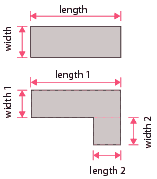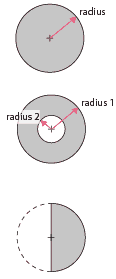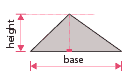# Quantity/Volume Calculators for Concrete (Imperial)

These calculators are intended to allow you to calculate the imperial volume of concrete required for paths etc.

Three calculators are provided, the first for rectangle areas of concrete, the second for circular areas and the final one for triangular areas.

## Rectangular Imperial volume calculator

 Enter the dimensions of the rectangle to be concreted: Length feet Width feet Thickness inches You will need approximately..... yds³ of concrete   Note: This is the volume of the work space and does not take into account the concrete 'shrinkage'For a simple rectangle, just use the length and width with the thickness in the calculator left.

For irregular, basically square areas,

• Breakdown the area into a number of simple rectangular areas.
• Workout the volume for each area separately.
• And finally add the separate volumes together.

## Circular Imperial volume calculator

 Enter the radius of the circle to be concreted: Radius feet Thickness inches You will need approximately..... yds³ of concrete   Note: This is the volume of the work space and does not take into account the concrete 'shrinkage'For a simple circle, just use the radius with the thickness in the calculator left.

For a ring:

• Use the calculator to work out the volume for both the inner and outer circles.
• Take the volume for the inner circle away from the volume for the outer circle and you will be left with the volume of the ring.

For part of a circle, calculate the volume for the full circle and then adjust for the part required - i.e. halve for a half circle, quarter for a quarter circle.

## Triangular Imperial volume calculator

 Enter the dimensions of the triangular areas to be concreted: Base feet Height feet Thickness inches You will need approximately..... yds³ of concrete ThiMessage Note: This is the volume of the work space and does not take into account the concrete 'shrinkage'For triangular shapes, work out the height and base of the triangle and use with the thickness in the calculator left.

The 'height' is the distance between an angle and the opposite side where a line meets the side at right angles. The side it meets is the 'base'.